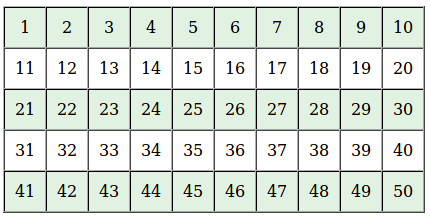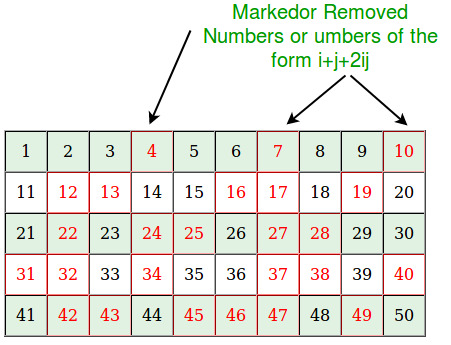GeeksforGeeks App
Open AppBrowser
Continue

## Related Articles

• Write an Interview Experience
• Mathematical Algorithms

# Sieve of Sundaram to print all primes smaller than n

Given a number n, print all primes smaller than or equal to n.

Examples:

```Input:  n = 10
Output: 2, 3, 5, 7

Input:  n = 20
Output: 2, 3, 5, 7, 11, 13, 17, 19```

We have discussed Sieve of Eratosthenes algorithm for the above task.

Below is Sieve of Sundaram algorithm.

```printPrimes(n)
[Prints all prime numbers smaller than n]

1) In general Sieve of Sundaram, produces primes smaller than
(2*x + 2) for given number x. Since we want primes
smaller than n, we reduce n-1 to half. We call it nNew.
nNew = (n-1)/2;
For example, if n = 102, then nNew = 50.
if n = 103, then nNew = 51

2) Create an array marked[n] that is going
to be used to separate numbers of the form i+j+2ij from
others where  1 <= i <= j

3) Initialize all entries of marked[] as false.

4) // Mark all numbers of the form i + j + 2ij as true
// where 1 <= i <= j
Loop for i=1 to nNew
a) j = i;
b) Loop While (i + j + 2*i*j)  2, then print 2 as first prime.

6) Remaining primes are of the form 2i + 1 where i is
index of NOT marked numbers. So print 2i + 1 for all i
such that marked[i] is false. ```

Below is the implementation of the above algorithm :

## C++

 `// C++ program to print primes smaller than n using``// Sieve of Sundaram.``#include ``using` `namespace` `std;` `// Prints all prime numbers smaller``int` `SieveOfSundaram(``int` `n)``{``    ``// In general Sieve of Sundaram, produces primes smaller``    ``// than (2*x + 2) for a number given number x.``    ``// Since we want primes smaller than n, we reduce n to half``    ``int` `nNew = (n-1)/2;` `    ``// This array is used to separate numbers of the form i+j+2ij``    ``// from others where  1 <= i <= j``    ``bool` `marked[nNew + 1];` `    ``// Initialize all elements as not marked``    ``memset``(marked, ``false``, ``sizeof``(marked));` `    ``// Main logic of Sundaram.  Mark all numbers of the``    ``// form i + j + 2ij as true where 1 <= i <= j``    ``for` `(``int` `i=1; i<=nNew; i++)``        ``for` `(``int` `j=i; (i + j + 2*i*j) <= nNew; j++)``            ``marked[i + j + 2*i*j] = ``true``;` `    ``// Since 2 is a prime number``    ``if` `(n > 2)``        ``cout << 2 << ``" "``;` `    ``// Print other primes. Remaining primes are of the form``    ``// 2*i + 1 such that marked[i] is false.``    ``for` `(``int` `i=1; i<=nNew; i++)``        ``if` `(marked[i] == ``false``)``            ``cout << 2*i + 1 << ``" "``;``}` `// Driver program to test above``int` `main(``void``)``{``    ``int` `n = 20;``    ``SieveOfSundaram(n);``    ``return` `0;``}`

## Java

 `// Java program to print primes smaller``// than n using Sieve of Sundaram.``import` `java.util.Arrays;``class` `GFG {` `// Prints all prime numbers smaller``static` `int` `SieveOfSundaram(``int` `n) {` `    ``// In general Sieve of Sundaram, produces``    ``// primes smaller than (2*x + 2) for a number``    ``// given number x. Since we want primes``    ``// smaller than n, we reduce n to half``    ``int` `nNew = (n - ``1``) / ``2``;` `    ``// This array is used to separate numbers of the``    ``// form i+j+2ij from others where 1 <= i <= j``    ``boolean` `marked[] = ``new` `boolean``[nNew + ``1``];` `    ``// Initialize all elements as not marked``    ``Arrays.fill(marked, ``false``);` `    ``// Main logic of Sundaram. Mark all numbers of the``    ``// form i + j + 2ij as true where 1 <= i <= j``    ``for` `(``int` `i = ``1``; i <= nNew; i++)``    ``for` `(``int` `j = i; (i + j + ``2` `* i * j) <= nNew; j++)``        ``marked[i + j + ``2` `* i * j] = ``true``;` `    ``// Since 2 is a prime number``    ``if` `(n > ``2``)``    ``System.out.print(``2` `+ ``" "``);` `    ``// Print other primes. Remaining primes are of``    ``// the form 2*i + 1 such that marked[i] is false.``    ``for` `(``int` `i = ``1``; i <= nNew; i++)``    ``if` `(marked[i] == ``false``)``        ``System.out.print(``2` `* i + ``1` `+ ``" "``);``    ``return` `-``1``;``}` `// Driver code``public` `static` `void` `main(String[] args) {``    ``int` `n = ``20``;``    ``SieveOfSundaram(n);``}``}``// This code is contributed by Anant Agarwal.`

## Python3

 `# Python3 program to print``# primes smaller than n using``# Sieve of Sundaram.` `# Prints all prime numbers smaller``def` `SieveOfSundaram(n):``    ` `    ``# In general Sieve of Sundaram,``    ``# produces primes smaller``    ``# than (2*x + 2) for a number``    ``# given number x. Since we want``    ``# primes smaller than n, we``    ``# reduce n to half``    ``nNew ``=` `int``((n ``-` `1``) ``/` `2``);` `    ``# This array is used to separate``    ``# numbers of the form i+j+2ij``    ``# from others where 1 <= i <= j``    ``# Initialize all elements as not marked``    ``marked ``=` `[``0``] ``*` `(nNew ``+` `1``);` `    ``# Main logic of Sundaram. Mark all``    ``# numbers of the form i + j + 2ij``    ``# as true where 1 <= i <= j``    ``for` `i ``in` `range``(``1``, nNew ``+` `1``):``        ``j ``=` `i;``        ``while``((i ``+` `j ``+` `2` `*` `i ``*` `j) <``=` `nNew):``            ``marked[i ``+` `j ``+` `2` `*` `i ``*` `j] ``=` `1``;``            ``j ``+``=` `1``;` `    ``# Since 2 is a prime number``    ``if` `(n > ``2``):``        ``print``(``2``, end ``=` `" "``);` `    ``# Print other primes. Remaining``    ``# primes are of the form 2*i + 1``    ``# such that marked[i] is false.``    ``for` `i ``in` `range``(``1``, nNew ``+` `1``):``        ``if` `(marked[i] ``=``=` `0``):``            ``print``((``2` `*` `i ``+` `1``), end ``=` `" "``);` `# Driver Code``n ``=` `20``;``SieveOfSundaram(n);` `# This code is contributed by mits`

## C#

 `// C# program to print primes smaller``// than n using Sieve of Sundaram.``using` `System;` `class` `GFG {` `// Prints all prime numbers smaller``static` `int` `SieveOfSundaram(``int` `n)``{` `    ``// In general Sieve of Sundaram, produces``    ``// primes smaller than (2*x + 2) for a number``    ``// given number x. Since we want primes``    ``// smaller than n, we reduce n to half``    ``int` `nNew = (n - 1) / 2;` `    ``// This array is used to separate``    ``// numbers of the form i+j+2ij from``    ``// others where 1 <= i <= j``    ``bool` `[]marked = ``new` `bool``[nNew + 1];` `    ``// Initialize all elements as not marked``    ``for` `(``int` `i=0;i 2)``    ``Console.Write(2 + ``" "``);` `    ``// Print other primes.``    ``// Remaining primes are of``    ``// the form 2*i + 1 such``    ``// that marked[i] is false.``    ``for` `(``int` `i = 1; i <= nNew; i++)``    ``if` `(marked[i] == ``false``)``        ``Console.Write(2 * i + 1 + ``" "``);``    ``return` `-1;``}` `// Driver code``public` `static` `void` `Main()``{``    ``int` `n = 20;``    ``SieveOfSundaram(n);``}``}` `// This code is contributed by nitin mittal`

## PHP

 ` 2)``        ``echo` `"2 "``;` `    ``// Print other primes. Remaining``    ``// primes are of the form 2*i + 1``    ``// such that marked[i] is false.``    ``for` `(``\$i` `= 1; ``\$i` `<= ``\$nNew``; ``\$i``++)``        ``if` `(``\$marked``[``\$i``] == false)``            ``echo` `(2 * ``\$i` `+ 1) . ``" "``;``}` `// Driver Code``\$n` `= 20;``SieveOfSundaram(``\$n``);` `// This code is contributed by mits``?>`

## Javascript

 ``

Output

`2 3 5 7 11 13 17 19 `

Time Complexity: O(n log n)
Auxiliary Space: O(n)

Illustration:
All red entries in below illustration are marked entries. For every remaining (or black) entry x, the number 2x+1 is prime.
Lets see how it works for n=102, we will have the sieve for (n-1)/2 as follows:Mark all the numbers which can be represented as i + j + 2ijNow for all the unmarked numbers in the list, find 2x+1 and that will be the prime:
Like 2*1+1=3
2*3+1=7
2*5+1=11
2*6+1=13
2*8+1=17 and so on..
How does this work?
When we produce our final output, we produce all integers of form 2x+1 (i.e., they are odd) except 2 which is handled separately.

```Let q be an integer of the form 2x + 1.

q is excluded if and only if x is of the
form i + j + 2ij. That means,

q = 2(i + j + 2ij) + 1
= (2i + 1)(2j + 1)

So, an odd integer is excluded from the final list if
and only if it has a factorization of the form (2i + 1)(2j + 1)
which is to say, if it has a non-trivial odd factor.

Source: Wiki```

Reference:
https://en.wikipedia.org/wiki/Sieve_of_Sundaram